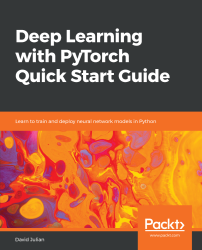•#### Deep Learning with PyTorch Quick Start Guide#### Overview of this book

PyTorch is extremely powerful and yet easy to learn. It provides advanced features, such as supporting multiprocessor, distributed, and parallel computation. This book is an excellent entry point for those wanting to explore deep learning with PyTorch to harness its power. This book will introduce you to the PyTorch deep learning library and teach you how to train deep learning models without any hassle. We will set up the deep learning environment using PyTorch, and then train and deploy different types of deep learning models, such as CNN, RNN, and autoencoders. You will learn how to optimize models by tuning hyperparameters and how to use PyTorch in multiprocessor and distributed environments. We will discuss long short-term memory network (LSTMs) and build a language model to predict text. By the end of this book, you will be familiar with PyTorch's capabilities and be able to utilize the library to train your neural networks with relative ease.
PrefaceFree Chapter
Introduction to PyTorchDeep Learning FundamentalsComputational Graphs and Linear ModelsConvolutional NetworksOther NN ArchitecturesGetting the Most out of PyTorchOther Books You May Enjoy# Models

Choosing a model representation is an important task in machine learning. So far, we have been referring to models as black boxes. Some data is put in, and, based on training, the model makes a prediction. Before we look inside this black box, let's review some of the linear algebra that we will need to understand deep learning models.

# Linear algebra review

Linear algebra is concerned with the representation of linear equations through the use of matrices. In the algebra taught in high school, we were concerned with scalar, that is, single number, values. We have equations, and rules for manipulating these equations, so that they can be evaluated. The same is true when, instead of scalar values, we use matrices...